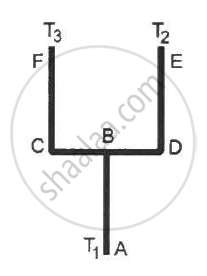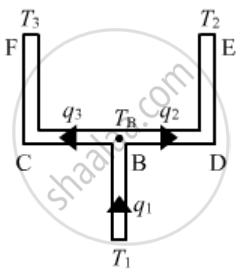Share

# Four Identical Rods Ab, Cd, Cf and De Are Joined as Shown in Following Figure . the Length, Cross-sectional Area and Thermal Conductivity of Each Rod Are L, a and K Respectively. - Physics

ConceptHeat Transfer Conduction

#### Question

Four identical rods AB, CD, CF and DE are joined as shown in following figure . The length, cross-sectional area and thermal conductivity of each rod are l, A and K respectively. The ends A, E and F are maintained at temperature T1, T2 and T3 respectively. Assuming no loss of heat to the atmosphere, find the temperature at B.#### SolutionLet the temperature at junction B be T.
Let q1q2 and q3 be the heat currents, i.e. rate of flow of heat per unit time in AB, BCE and BDF, respectively.
From the diagram, we can see that
q1 = q2 + q3
The rate of flow of heat is given by
q = (KA DeltaT)/l
Using this tn the above equation, we get
(A (T_1 - T))/l = (KA  ( T + T_3))/(31/2)  + (KA (T-T_2))/(31/2)

⇒ T_1 - T = (2(T  - T_3))/3+(2(T- T_2))/3

⇒ 3 (T1 - T) = 2T - 2T3 + 2T - 2T2

⇒ T = (-3T_1 + 2( T_2 +T_3))/7

Is there an error in this question or solution?

#### APPEARS IN

Solution Four Identical Rods Ab, Cd, Cf and De Are Joined as Shown in Following Figure . the Length, Cross-sectional Area and Thermal Conductivity of Each Rod Are L, a and K Respectively. Concept: Heat Transfer - Conduction.
S